×

×

PSU / Engineering / CS 250 / What is the meaning of adjacent nodes?

# What is the meaning of adjacent nodes? Description

##### Description: Here are my notes for week 6, 7, 9 and 10. My week 8 notes are attached to the second midterm and weeks 1-5 are attached to the first midterm. Hope these help and good luck!
19 Pages 52 Views 2 Unlocks
Reviews

CS250, Week 10 Notes

## What is the meaning of adjacent nodes?PSU: Fall term

Graph Theory:

A graph is a pair (V, E), where V is a set of elements called vertices, nodes, or points  and E is a set of pairs of vertices called edges or arcs.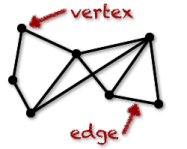Variations:

Edges can be directed or undirected. Don't forget about the age old question of What is the purpose of the treasury dept in the federal government?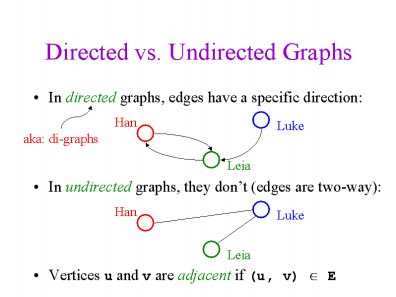## What is the meaning of isolated vertex?Edges can be weighted.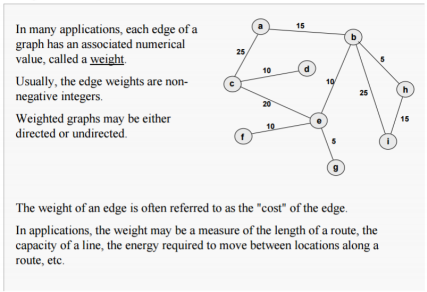Edges can cycle:Don't forget about the age old question of What is the meaning of pareto improvement in economics?

Notations:

## What is the meaning of the adjacency matrix?Initial and terminal node of an edge

A digraph (or a directed graph) is a graph in which the edges are directed. (Formally:  a digraph is a (usually finite) set of vertices V and set of ordered pairs (a,b) (where a,  b are in V) called edges. The vertex a is the initial vertex of the edge  and b the terminal vertex. We also discuss several other topics like What is the formula of derivatives?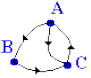Edge incident to and from a node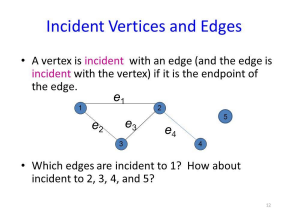Degrees of vertex, isolated (0), pendant (1)

A vertex with degree zero is called an isolated vertex.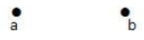By using degree of a vertex, we have a two special types of vertices. A vertex  with degree one is called a pendent vertex.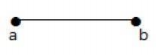Don't forget about the age old question of Which manner of articulation refers to the restriction of airflow in the oral cavity thus causing turbulence and noise?

In-degree deg-(v) and out degree deg+(v) of node v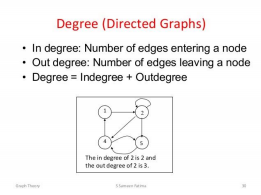Don't forget about the age old question of Who is shot and killed by mark chapman in 1980?

In graph theory and computer science, an adjacency matrix is a square matrix used to  represent a finite graph. The elements of the matrix indicate whether pairs of vertices are  adjacent or not in the graph. In the special case of a finite simple graph, the adjacency  matrix is a (0,1)-matrix with zeros on its diagonal.We also discuss several other topics like What was the observed sample mean breaking strength for the 49 randomly selected bags?

Incidence matrix:

In mathematics, an incidence matrix is a matrixthat shows the relationship between two  classes of objects. If the first class is X and the second is Y, the matrix has one row for each  element of X and one column for each element of Y.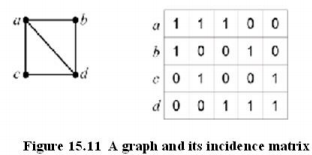Every node stores a list of adjacent nodes

In graph theory and computer science, an adjacency list is a collection of unordered lists used  to represent a finite graph. Each list describes the set of neighbors of a vertex in the graph.

Graph Isomorphism:

Two graphs which contain the same number of graph vertices connected in the same way are  said to be isomorphic. Formally, two graphs and with graph vertices are said to  be isomorphic if there is a permutation of such that is in the set of graph edges iff is in the set  of graph edges .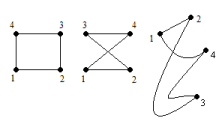Paths and Circuits:

Path: a sequence of nodes (edges) that begins at a vertex and travels from vertex to  vertex along edges of the graphCircuit: a trail which starts and ends at the same vertex

Simple:Connected graph: when there is a path between every pair of vertices (nodes)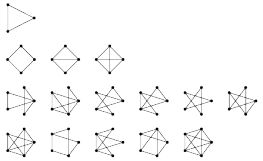Euler path/circuit: traverses every arc exactly once

Hamilton path/circuit: contains every node exactly once

Binary relations: A directed graph defines a binary relation on a set of vice versa.  Properties are easier to see on a graph and closures easier to compute.

Spanning trees: Traversal: list al nodes only once.

Depth-first: go as far as possible, come back to last choice, take another path  RESULT: ABDFECG

Breadth-first: all 1 step, all 2 steps, all 3 steps… RESULT: ABCDEFG Minimal spanning tree:

Prim (greedy): add cheapest edge from tree so far to outside nodes.  Kruskal: add cheapest edge to bridge disconnected subtrees.

Reference Page:

CS250, Week 9 Notes

PSU: Fall term

Decision trees:

Definition: A node is a question, an edge is an answer. Leafs are the outcomes.

Huffman Codes:

Maps characters to bit strings so that more frequent characters are mapped to shorter  strings.

The idea is to assign variable-length codes to input characters, lengths of the assigned  codes are based on the frequencies of corresponding characters.

Traversal:

Polish notation: Way of expressing arithmetic expressions that avoids the use of  brackets to define priorities for evaluation of operators.

Ex. (3 + 5) * (7 – 2)

In polish notation… * + 3 5 – 7 2

Tree representation of expressions:

Preorder: Visit the root, traverse to the left subtree, traverse the right subtree

Postorder: traverse the left subtree, traverse right subtree, visit root

Inorder (only for binary): Traverse the left subtree, visit the root, traverse the right  subtree

Reference Page:

CS250, Week 7 Notes

PSU: Fall term

Trees: A tree is a mathematical structure that can be viewed as either a graph or as  a data structure. The two views are equivalent, since a tree data structure contains not  only a set of elements, but also connections between elements, giving a tree graph.

Binary tree: a data structure in which a record is linked to two successor records,  usually referred to as the left branch when greater and the right when less than the  previous record.

Reference Page:

CS 250, Week 6 Notes

PSU: Fall term

Cantor Diagonalization: Cantor diagonal argument is an argument to prove that set  of real numbers is uncountable.

What is a countable set? Let's say a set is countable if we can start ordering the elements of a  set like the first, the second and so on. Formally we have to find a bijection with natural  numbers.

To prove that reals are uncountable we first assume the contrary, namely that set of reals is  countable. Then we have to find a contradiction, rendering the assumption false. To do that  we find a real number which is not counted. Cantor diagonal argument construct such a real  number which is not counted.

So here are the steps:

Goal: Set of real numbers is uncountable.

Step 1: Assume that the set is countable. This means that the set of real numbers can be  written as a set with first element, second element and so on, which

is {r1,r2,r3,…}{r1,r2,r3,…}.

Step 2: One way to show that the assumption of step 2 is not possible is to find a real  number which is not counted there. How? By Cantor diagonal argument. Suppose that we are going to consider only numbers between 0 and 1. Then the new number  is such that it is different from the first number at the first digit, from the second element at  the second digit and so on. For instance look at the following:

0 9 7 0 6

0 8 2 4 3

0 4 5 2 8

0 1 2 5 3

0 8 1 1 4

You can see that the number is different from all counted numbers and also you can see that it is  constructed by using the diagonal elements of counted numbers written as above, hence the  nominalization of Diagonal argument.

Permutation: An argument of k objects from a set of n.

No repetitions, order matters.

Permutation is all possible ways of doing something without any repetitions.

Combinations: Order doesn’t matter.

Pigeonhole Principle: If n pigeonholes are occupied by m pigeons and m>n, then at least one pigeonhole is occupied by more than one pigeon.

Probability Function: S is a set called space whose elements are called points. A subset E of S is called an event.